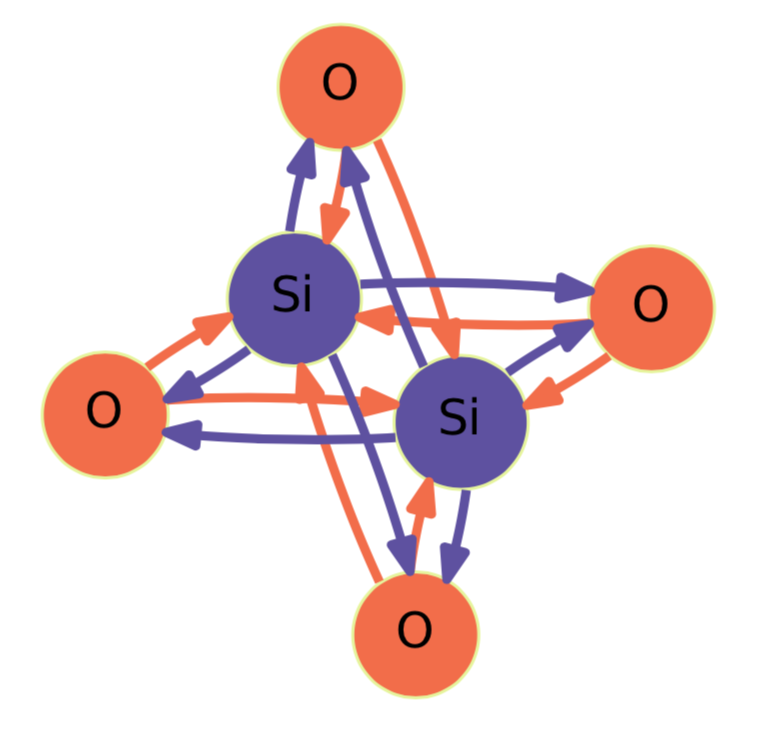# Crystal Graph Neural Networks

This repository contains the original implementation of the CGNN architectures described in the paper "Crystal Graph Neural Networks for Data Mining in Materials Science".Gilmer, et al. investigated various graph neural networks for predicting molecular properties, and proposed the neural message passing framework that unifies them. Xie, et al. studied graph neural networks to predict bulk properties of crystalline materials, and used a multi-graph named a crystal graph. Schütt, et al. proposed a deep learning architecture with an implicit graph neural network not only to predict material properties, but also to perform molecular dynamics simulations. These studies use bond distances as features for machine learning. In contrast, the CGNN architectures use no bond distances to predict bulk properties at equilibrium states of crystalline materials at 0 K and 0 Pa, such as the formation energy, the unit cell volume, the band gap, and the total magnetization.

Note that the crystal graph represents only a repeating unit of a periodic graph or a crystal net in crystallography.

## Requirements

• Python 3.7
• PyTorch 1.0
• Pandas
• Matplotlib (necessary for plotting scripts)

## Installation

``````git clone https://github.com/Tony-Y/cgnn.git
CGNN_HOME=`pwd`/cgnn
``````

## Usage

The user guide in this GitHub Pages site provides the complete explanation of the CGNN architectures, and the description of program options. Usage examples are contained in the directory `cgnn/examples`.

### Dataset Files

The CGNN code needs the following files:

• `targets.csv` consists of all target values.
• `graph_data.npz` consists of all node and neighbor lists of graphs.
• `config.json` defines node vectors.
• `split.json` defines data splitting (train/val/test).

#### Target Values

`targets.csv` must have a header row consisting `name` and target names such as `formation_energy_per_atom`, `volume_deviation`, `band_gap`, and `magnetization_per_atom`. The `name` column must store identifiers like an ID number or string that is unique to each example in the dataset. The target columns must store numerical values excluding `NaN` and `None`.

#### Crystal Graphs

You can create a graph data file (`graph_data.npz`) as follows:

```graphs = dict()
for name, structure in dataset:
nodes = ... # A species-index list
neighbors = ... # A list of neighbor lists
graphs[name] = (nodes, neighbors)
np.savez_compressed('graph_data.npz', graph_dict=graphs)
```

where `name` is the same identifier as in `targets.csv` for each example.

`tools/mp_graph.py` creates graph data from structures given in the Materials Project structure format. This tool is used when the OQMD dataset is compiled.

#### Node Vectors

You can create a configuration file (`config.json`) using the one-hot encoding as follows:

```n_species = ... # The number of node species
config = dict()
config["node_vectors"] = np.eye(n_species,n_species).tolist()
with open("config.json", 'w') as f:
json.dump(config, f)
```

#### Data Splitting

You can create a data-splitting file (`split.json`) as follows:

```split = dict()
split["train"] = ... # The index list for the training set
split["val"] = ... # The index list for the validation set
split["test"] = ... # The index list for the testing set
with open("split.json", 'w') as f:
json.dump(split, f)
```

where the index, which must be a non-negative integer, is a row label of the data frame that the CSV file `targets.csv` is read into.

### Training

A training script example:

```NodeFeatures=... # The size of a node vector
DATASET=\${CGNN_HOME}/YourDataset
python \${CGNN_HOME}/src/cgnn.py \
--num_epochs 100 \
--batch_size 512 \
--lr 0.001 \
--n_node_feat \${NodeFeatures} \
--n_hidden_feat 64 \
--n_graph_feat 128 \
--n_conv 3 \
--n_fc 2 \
--dataset_path \${DATASET} \
--split_file \${DATASET}/split.json \
--target_name formation_energy_per_atom \
--milestones 80 \
--gamma 0.1 \
```

You can see the training history using `tools/plot_history.py` that plots the root mean squared errors (RMSEs) and the mean absolute errors (MAEs) for the training and validation sets. The values of the loss (the mean squared error, MSE) and the MAE are written to `history.csv` for every epoch.

```python \${CGNN_HOME}/tools/plot_history.py
```

After the end of the training, predictions for the testing set are written to `test_predictions.csv`. You can see the predictions compared to the target values using `tools/plot_test.py`.

```python \${CGNN_HOME}/tools/plot_test.py
```

### Prediction

The prediction for new data is conducted using the testing-only mode of the program. You first prepare a new dataset with a testing set including all examples to be predicted. The prediction configuration must have all the same parameters as the training configuration except for the total number of epochs, which must be zero for testing only. In addition, you must specify the model to be loaded using `--load_model YourModel`.

```DATASET=\${CGNN_HOME}/NewDataset
python \${CGNN_HOME}/src/cgnn.py \
--num_epochs 0 \
--batch_size 512 \
--lr 0.001 \
--n_node_feat \${NodeFeatures} \
--n_hidden_feat 64 \
--n_graph_feat 128 \
--n_conv 3 \
--n_fc 2 \
--dataset_path \${DATASET} \
--split_file \${DATASET}/split.json \
--target_name formation_energy_per_atom \
--milestones 80 \
--gamma 0.1 \
--load_model \${MODEL} \
```

## The Open Quantum Materials Database

The OQMD v1.2 contains 563k entries, and is available from the OQMD site. The detail setup of the database is described in the README in the directory `cgnn/OQMD`.

## Citation

When you mention this work, please cite the CGNN paper:

``````@techreport{yamamoto2019cgnn,
Author = {Takenori Yamamoto},
Title = {Crystal Graph Neural Networks for Data Mining in Materials Science},
Address = {Yokohama, Japan},
Institution = {Research Institute for Mathematical and Computational Sciences, LLC},
Year = {2019},
Note = {https://github.com/Tony-Y/cgnn}
}
``````

## References

Get A Weekly Email With Trending Projects For These Topics
No Spam. Unsubscribe easily at any time.
python (51,962
pytorch (2,279
neural-networks (425
data-mining (165
graph-theory (28
materials-science (16